##### Search### KCSE CLUSTER TESTS 15

#### Chemistry Paper 3

1.

Volume of pipette. 25.0 cm3 Penalize ½ mark if not in decimal Table 1 (3marks)

— Complete table 1 mark√1

Conditions:

-Complete table with 3 titration 1 mark√1

-Incomplete table with 1 titration = 0 mark

— use of decimals (tied to rows 1 and 2 only ) = 1 mark)√1

Conditions:

-Accept only 1 to 2 decimal place used consistently; otherwise penalize fully.

-Accept 2 decimal place if the second dp is ‘0’ ‘5’ otherwise penalize fully.

-Accept inconsistency use of zeros as initials volume i.e 0,0.0,0.00.

— Accuracy = 1 mark (only tick the correct value otherwise don’t tick);

-Compare the candidates /students value with the school value (S.V): and tick (√) the chosen value where it earns a mark.√1

Conditions: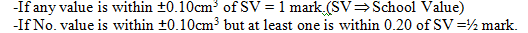-If no value is within 0.20 cm3 of SV = 0 mark.

Note:

-If there is wrong arithmetic//subtraction in the table compare the SV with the worked out Correct value// the titre and award accordingly.

— principal of averaging = 1 mark√1 values averaged MUST be shown and MUST be within ±0.20 cm3 of each other,

Conditions:

-If three consistent values are averaged. = √1

-If 3 titrations are done but only 2 are within 0.20cm3 of each other rare averaged = 1 mark.

-If only 2 titrations are done, are consistent and are averaged =½ mark.

-If only 2 titrations done, are inconsistent and get averaged = 0 mark.

Penalties:

-Penalize ½ mark for wrong arithmetic error outside +2 units in the second decimal place.

-Penalize½ mark if no working is shown but the answer is correct.

-If no working is shown and the answer is wrong, penalize fully.

-Accept rounding off the answer to 2 dp. Otherwise penalize ½ mark for wrong rounding off to whole numbers.

Note

-Accept answer if it works out exactly to 1 dp as to a whole number and credit fully.

— Final answer (tied to correct average titre) = 1 mark.√1

-Compare the students//candidates correct average titre to the SV then: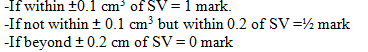Note

-If wrong values are averaged or when there are no options for averaging pick the correct Values (if any) following the principle of averaging average and award accordingly.

Examples

-For 24.9, 24.7 and the SV is 24.35 pick 24.6 and 24.7.

-For 24.2,24.4 and 24.3 pick all.

-For 25.3,25.1 and 25.5 and the SV is 24.5 pick either 25.3 and 25.1 or 25.3 and 25.5 depending in the SV but not all.

-For 24.6,24.3 and 24.9 none to be picked for averaging and award 0 mark for final answer.

Procedure II

Calculations:(Questions and calculations)

(a) The average titre = 25.0 cm3

Principles of averaging = 1 mark

-Values averaging MUST be shown and MUST be within ±0.20cm3 of each other.

Conditions:

-If three consistent values are averaged = 1 mark√1

-If 3 titrations are done but only 2 are within 0.20 cm3 of each other are averaged = 1 mark√1

-If only 2 titrations are done, are consistent and are averaged = ½ mark.

-If 3 possible but only 2 re-arranged = 0 mark.

-If 3 titrations are done, are inconsistent and yet are ALL averaged = 0 mark.

-if only 2 titrations done, are inconsistent and yet averaged = 0 mark.

Penalties:

-Penalise½ mark for wrong arithmetic error outside +2 and in the second decimal place.

-Penalize ½ mark if no working is shown but the answer is correct.

-If no working is shown and the answer is wrong, penalize fully;

-Accept rounding off the answer to 2 decimal places; otherwise penalize ½ mark for wrong rounding off and 1 dp or to whole numbers.

Note:

-Accept answers if it works out exactly to 1 dp a whole number and credit fully.

(b). Moles of HA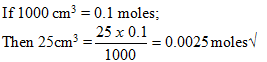Conditions and penalties

-Penalize ½ mark for wrong transfer of molarity otherwise penalize fully.

-Accept rounding of answer to at least 3 dp. otherwise penalize ½ mark.

-Penalise ½ mark for wrong answer if the arithmetic error is outside ±2 units in the 3 dp.

-Units given must be correct; penalize ½ mark for wrong units.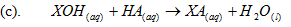(d). From moles ration: 1 mole of acid reacts with 1 mole of alkali;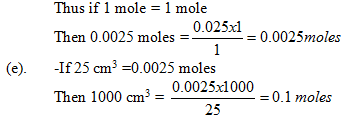Conditions and penalties:

-Penalize ½ mark for wrong transfer of moles, otherwise penalize fully.

-Accept rounding off of answers to atleast 3 dp otherwise penalize ½ mark.

-Penalise ½ mark for wrong answer if the arithmetic error is outside ± 2 units in the 3 dp.

-Units given must be correct; penalize ½ mark for wrong units..

(ii). Mass = moles /litre x RMM.

Conditions and penalties:

-Penalize½ mark for wrong transfer of molarity and mass; otherwise penalize fully.

-Accept rounding off of answer to at least 3 dp. otherwise penalize ½ mark.

- Penalize ½ mark for wrong answer if the arithmetic error is outside ± 2 units in the 3 dp.

-Units given must be correct. Penalize ½ mark for wrong units.

(f). Concentration of XCl

Total mass – mass of KoH

8.4g – 5.6 = 2.8g/litre.

Conditions and penalties:

-Penalize ½ mark(½√) for wrong transfer of answers in (ii) above. Otherwise penalize fully.

-Accept rounding off of answers to atleast 3 dp. otherwise penalize √½ mark.

-Penalize ½ mark for wrong answers if the arithmetic error is outside ±2 units in the 3 dp.

-Units given must be correct; penalize ½ mark for wrong units.

(g). Percentage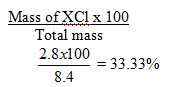Conditions

-Penalize ½ mark for wrong transfer of answers in f above. otherwise penalize fully.

-Accept rounding off to answer to atleast 3 dp. otherwise penalize ½ mark.

-Penalize ½ mark for wrong answer if the arithmetic error is outside ± 2 units in the 3 dp.

-Units given must be correct; penalize ½ mark for wrong units.

22 marks

2.

Complete table.

(a). Complete table√1

Conditions:

Complete table with 3 titration = √1

Incomplete table with 2 titration =√½

Incomplete table with 1 titration = 0 mark

Penalties

-Wrong arithmetic// subtraction.

-Inverted tables.

-Burette readings beyond 50.0 cm3, unless explained.

-unrealistic litre values i.e too low (below 1.0 cm3) or too high (100cm3)

Note

-Penalized √½ mark EACH to a maximum penalty of √ mark (i.e) penalize ½ mark only once.

(b). Use of decimal (tied to rows 1 and 2 only) = 1 mark.

Conditions

-Accept only 1 to 2 dp used consistently; otherwise penalize fully.

-Accept 2 dp only if the second dp is ‘0’ ‘5’ otherwise penalize fully.

-Accuracy = 1 mark (only tick the correct value i.e 0,0.0,0.00

(c). Accuracy = 1 mark (only tick the correct value otherwise don’t tick.

-Compare the candidates//students value with school value (SV), and tick (√) the chosen value where it earns a mark.

Conditions

-If any value is within ± 0.10 cm3 of SV award √1

-If no value is within ± 0.10 cm3 but atleast one is within 0.20 of SV =½ mark (√½)

-If no value is within 0.20cm3 of SV award zero mark (0 mark)

Note:

If there is wrong arithmetic //substracha in the table compare the SV with the worked out correct value// the tilre and award accordingly.

(b).

i. N

ii. R

iii. P

iv. M

(c). (i). Sample M

9 marks

3.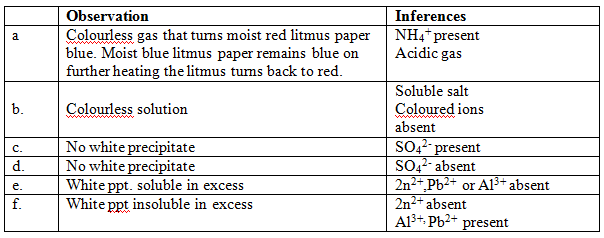9 marks

Back Top